instrumentation, inertial navigation, and in many other applications to detect and measure angular rates of change. A rate gyro (sometimes called a rate-of-turn gyro) consists of a spinning rotor mounted in a single gimbal, as shown in figure 3-13. A gyro mounted in this manner has one degree of freedom; that is, it is free to tilt in only one direction. ">Custom SearchRATE GYROS RATE GYROS are used in weapons control equipment, aircraft instrumentation, inertial navigation, and in many other applications to detect and measure angular rates of change. A rate gyro (sometimes called a rate-of-turn gyro) consists of a spinning rotor mounted in a single gimbal, as shown in figure 3-13. A gyro mounted in this manner has one degree of freedom; that is, it is free to tilt in only one direction. The rotor in a rate gyro is restrained from precessing by some means, usually a spring arrangement. This is done to limit precession and to return the rotor to a neutral position when there is no angular change taking place. Remember, the amount of precession of a gyro is proportional to the force that causes the precession. Figure 3-13. - Rate gyro (single degree of freedom).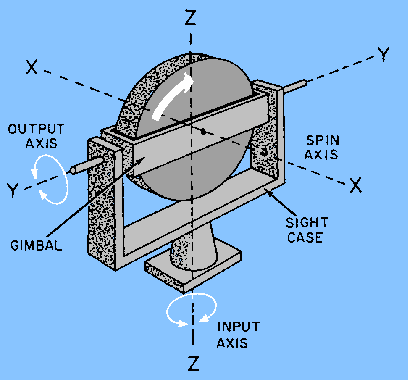If you attempt to change the gyro's plane of rotor spin by rotating the case about the input axis, the gyro will precess as shown in figure 3-14. From what you learned earlier in this chapter, the gyro does not appear to be obeying the rules for precession. However, turning the gyro case has the same effect as applying a torque on the spin axis. This is illustrated by arrow F in figure 3-14. You can determine the direction of precession by using the right-hand rule we discussed earlier. Figure 3-14. - Rate gyro precession.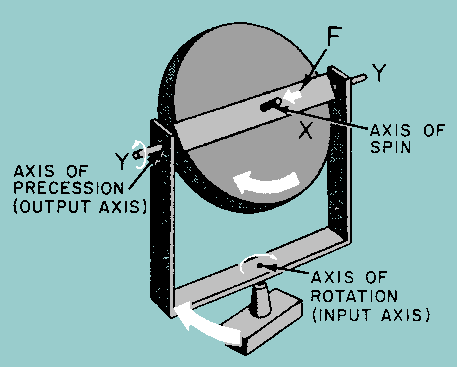The force applied at F will cause the gyro to precess at right angles to the force. Likewise, attempting to turn the gyro case will cause the same result. The gyro will precess, as shown by the arrows, around the Y-Y axis (output axis). Since the rate of precession is proportional to the applied force, you can increase the precession by increasing the speed with which you are moving the gyro case. In other words, you have a rate gyro. The faster you turn the case, the more the gyro will precess, since the amount of precession is proportional to the rate at which you are turning the gyro case. This characteristic of a gyro, when properly used, fits the requirements needed to sense the rate of motion about any axis. Figure 3-15 shows a method of restraining the precession of a gyro to permit the calculation of an angle. Springs have been attached to the crossarm of the output shaft. These springs restrain the free precession of the gyro. The gyro may use other types of restraint, but no matter what type of restraint is used, the gyro is harnessed to produce some useful work. Figure 3-15. - Precession of a spring restrained rate gyro.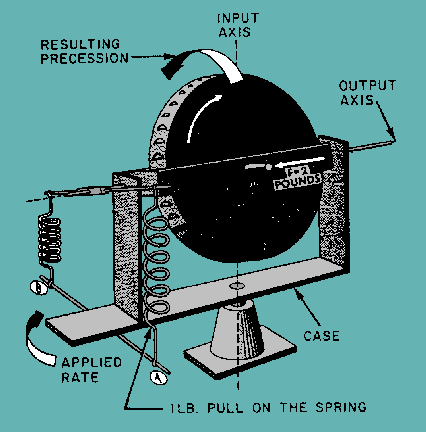As the gyro precesses, it exerts a precessional force against the springs that is proportional to the momentum of the spinning wheel and the applied force. For example, suppose you rotate the gyro case (fig. 3-15) at a speed corresponding to a horizontal force of 2 pounds at F. Obviously, the gyro will precess; and as it does, it will cause the crossarm to pull up on spring A with a certain force, say 1 pound. (This amount of force would vary with the length of the crossarm.) If you continue to turn the gyro case at this rate, the precession of the gyro will continually exert a pull on the spring. More precisely, the gyro will precess until the 1 pound pull of the crossarm is exactly counterbalanced by the tension of the spring; it will remain in a fixed position, as shown in figure 3-15. That is, it will remain in the precessed position as long as you continue to rotate the gyro case at the same, constant speed. A pointer attached to the output axis could be used with a calibrated scale to measure precise angular rates. When you stop moving the case, you remove the force at F, and the gyro stops precessing. The spring is still exerting a pull, however, so it pulls the crossarm back to the neutral position and returns the pointer to "zero." Suppose you now rotate the gyro case at a speed twice as fast as before, and in the same direction. This will be equal to a 4-pound force applied at F and a resulting 2-pound pull by the crossarm on spring A. In this situation the gyro will precess twice as far before the tension on the restraining spring equals the pull on the crossarm. Precession increases when the rate of rotation increases, as shown in figure 3-16. Figure 3-16. - Precession is proportional to the rate of rotation.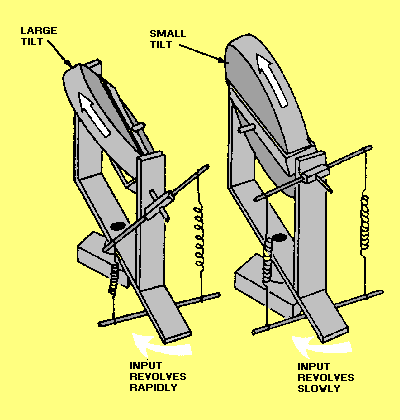Another type of rate gyro (often used in inertial navigation equipment) is the floated gyro unit. This unit generally uses a restraint known as a torsion bar. The advantage of the torsion bar over the spring is that the torsion bar needs no lever arm to exert torque. The torsion bar is mounted along the output axis (fig. 3-17), and produces restraining torque in either direction by twisting instead of pulling. Also, there is no gimbal bearing friction to cause interference with gyro operation. Figure 3-17. - Torsion bar-restrained floated rate gyro.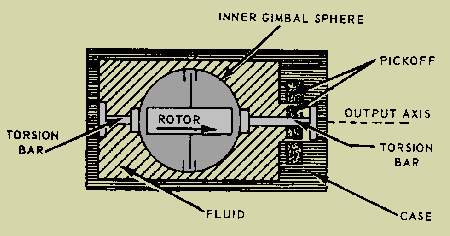A fluid surrounds the gyro sphere and provides flotation. It also provides protection from shock, and damps the oscillations resulting from sudden changes in the angular rate input. In this gyro, the inner gimbal displacement must be measured with some type of electrical pickoff. As the gyro case is rotated about the input axis, clockwise or counterclockwise, a precession torque will be developed about the output axis that will cause the inner gimbal to exert torque against the torsion bars. The torsion bars provide a restraining torque proportional to the amount of the inner gimbal's displacement. When the exerted gimbal torque is exactly opposed by the restraining torque provided by the torsion bars, the inner gimbal displacement will be proportional to the rate of rotation of the gyro case about the input axis. The pickoff measures this displacement and provides a signal whose amplitude and polarity (or phase) represent the direction and magnitude of the input angular velocity. The important point to remember is that every "rate" gyro measures the RATE OF ROTATION ABOUT ITS INPUT AXIS. Up to this point, we have illustrated only basic gyros. We used these basic, or simple, gyros to explain their principles of operation In actuality, the rate gyros used in typical modern day weapon systems are considerably more complex, and in some cases, very compact. Figure 3-18 shows a cutaway view of a rate gyro used in our Navy's missile systems and aircraft. Figure 3-18. - Rate gyro, cutaway view.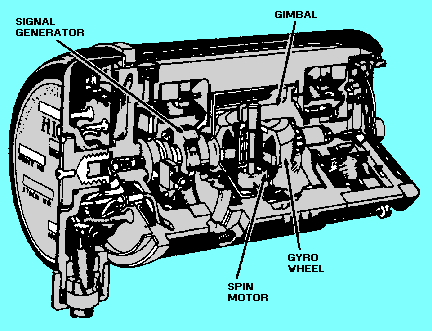Q.18 What are rate gyros primarily used for?Q.19 How many degrees-of-freedom does a rate gyro usually have?Q.20 What gyro characteristic provides the basis of the operation of a rate gyro?Privacy Statement - Copyright Information. - Contact Us